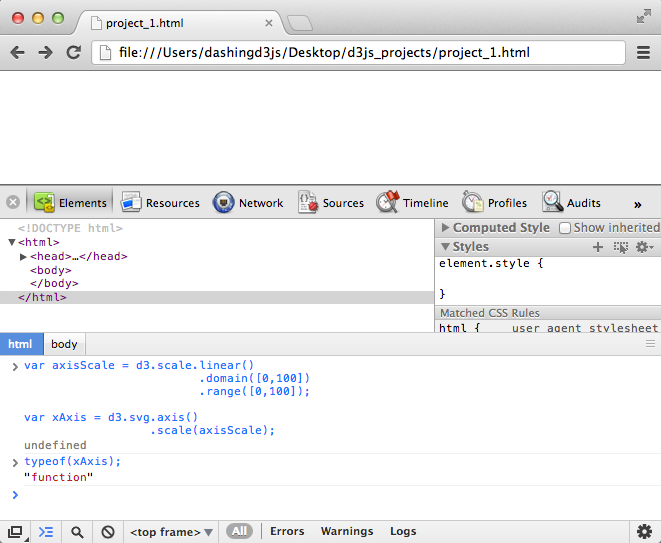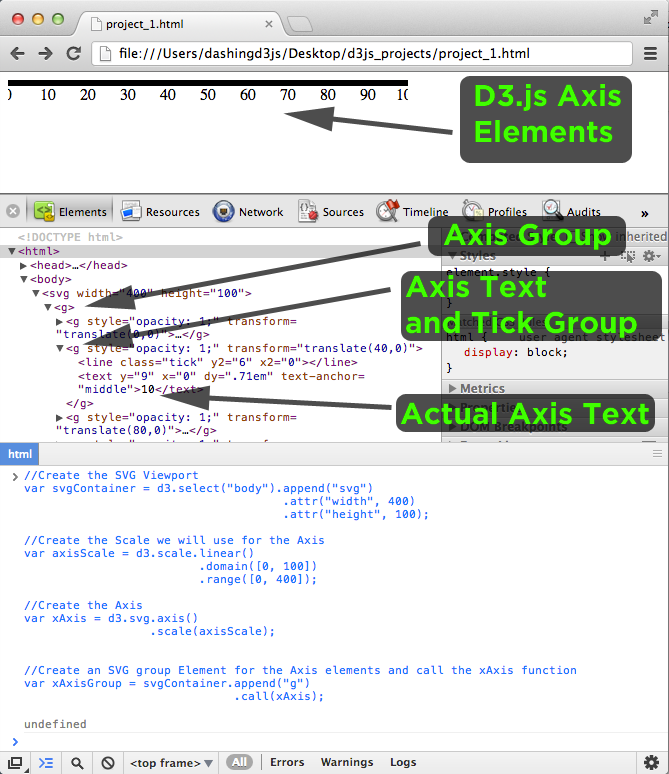# D3.js Axes

### The Goal

In this section, we will cover the D3.js Axis Component, why it is important and how we use it within our D3.js Data Visualizations.

First, we will cover what the D3.js Axis Component is and how we will use it.

Then, we will generate the D3.js Axis Component to get a feel for how it create one.

### D3.js Axis Component

Given a data set and D3.js Scales, the D3.js Axis component allows for easy addition of a horizontal-axis and vertical-axis to any graph.

The D3.js Axis Component displays reference lines for D3.js Scales automatically.

D3.js figures out for you how to draw the vertical axis line, the vertical axis ticks, the correct spacing of axis ticks to make the axis visually appealing and many other things.

D3.js also figures out for you how to draw the horizontal axis line, the horizontal axis ticks, the correct spacing to make the axis visually appealing and may other things.

From the earliest math classes, we were told to add axes and labels to our graphs.

So of course we will add axes and labels to our graphs and data visualizations.

But why?

The reason comes from the two reasons we use graphs:

• To show a relationships between variables
• To have the relationship be understood without explanation

In order to quickly ascertain the right relationship between the data represented, it is useful to have axes.

These axes allow us to interpret the relationship between variables.

In the SVG Circle example for instance:

```1<svg width="100" height="100">
2  <circle cx="20" cy="20" r="20" fill="green" />
3  <circle cx="70" cy="70" r="20" fill="purple" />
4</svg>```

Is it easy to interpret the relationship between the two circles?

No.

In normal math coordinates, the purple circle appears lower than the green circle, so we would guess that as the left/right variable moves left to right, the up/down variable would move down.

However, as we are in the SVG coordinate space, what appears to be happening is that as the left/right variable moves left to right, the up/down variable moves up.

As it moves up, is it a linear relationship, logarithmic relationship, or is the graph already inverted so it's actually moving down, or....?

As you can see/think/imagine, without axes, it is very hard to understand the relationship between the variables.

### Horizontal Axis and Vertical Axis

A large portion of the charts and graphs we build (and will cover) using D3.js contain a Horizontal Axis and a Vertical Axis.

For ease of exposition and brevity, we will call the Horizontal Axis the x-axis and the Vertical Axis the y-axis.

When we use the D3.js Axes Component, the axis function will return lines, ticks and labels.

We will refer to this mix of lines, ticks and labels as the axis.

When we talk about the x-axis and y-axis, we mean the combined visual elements that represent the horizontal and vertical axis.

### Scale of Axis

Before we get into the axis position, axis labels, axis lines and axis tickmarks, we will talk about the scale of the axis first.

The Scale of the Axis tells us the following information:

• The minimum number
• The maximum number
• Whether the scale is inverted
• What type of Scale we are dealing with:
• Quantitative
• Time
• Ordinal
• The units of the variable involved
• Etc...

Luckily for us, D3.js provides a way to tell our axis all of this information out of the box.

If you recall the D3.js Scales section, we covered how D3.js provides functions to perform data transformations.

The functions are specific by d3.scales, allowing us to write the following:

```1var axisScale = d3.scale.linear()
2                         .domain([0,100])
3                         .range([0,100]);```

D3.js allows us to pass this scale function to the axis function in order to provide all of the information needed to properly construct the axis.

### Generating a D3.js Axis

To generate the simplest D3.js Axis, we type:

`1var xAxis = d3.svg.axis();`

The axis variable is named "xAxis" for convenience, you can name the variable what you like.

We can then scale the default axis by passing the scale variable to the scale function:

```1var axisScale = d3.scale.linear()
2                         .domain([0,100])
3                         .range([0,100]);
4
5var xAxis = d3.svg.axis()
6                  .scale(axisScale);
7
8typeof(xAxis);```

Note - we add the typeof(xAxis); JavaScript to get a feel for what the xAxis variable actually is...

When we type the above into the JavaScript console, we get the following:This shows us that we have created and updated the parameters of a "function".

In case you are not familiar with the typeof JavaScript function:

`1typeof();`

The "typeof" operator in JavaScript lets you to probe the data type of its operand, such as whether a variable is function, object, string, numeric, or even undefined.

### Calling the D3.js Axis Function

Why is it important that the D3.js axis is a function?

It is important, because we have to call the axis function, passing in a current selection along with any optional arguments.

This custom control flow is the same as invoking the axis function by hand, but it makes it easier for method chaining.

It is worth noting that the call operator always returns the current selection.

To add the axis to the SVG graph, we will have to call the axis function on a selection.

Let us generate the basic SVG viewport and use that as our selection:

``` 1//Create the SVG Viewport selection
2var svgContainer = d3.select("body").append("svg")
3                                     .attr("width", 400)
4                                     .attr("height", 100);
5
6//Create the Scale we will use for the Axis
7var axisScale = d3.scale.linear()
8                         .domain([0, 100])
9                         .range([0, 400]);
10
11//Create the Axis
12var xAxis = d3.svg.axis()
13                  .scale(axisScale);```

Three things to note before we go on:

1. The width of the SVG Viewport is 400 units
2. We want to map the numbers of 0 to 100 (.domain([0, 100]))
onto the width of the view port - which is 400 units
3. So we define the range of the scale as .range([0, 400])

Now that we have that, we use the .call() operator to call the axis function:

```1//Create a group Element for the Axis elements and call the xAxis function
2var xAxisGroup = svgContainer.append("g")
3                              .call(xAxis);```

We create an SVG Group Element to hold all the elements that the axis function produces.

This makes it easier to transform, translate, and style the group as a whole.

After we append the group element, we call the xAxis function on the selection.

So if we type everything into the JavaScript Console:

``` 1//Create the SVG Viewport
2var svgContainer = d3.select("body").append("svg")
3                                     .attr("width", 400)
4                                     .attr("height", 100);
5
6//Create the Scale we will use for the Axis
7var axisScale = d3.scale.linear()
8                         .domain([0, 100])
9                         .range([0, 400]);
10
11//Create the Axis
12var xAxis = d3.svg.axis()
13                   .scale(axisScale);
14
15
16//Create an SVG group Element for the Axis elements and call the xAxis function
17var xAxisGroup = svgContainer.append("g")
18                              .call(xAxis);```

We get:Brilliant!

A couple of things to notice:

• The axis was created at the top of the SVG Viewport
• All of the Axis elements were created inside of the SVG Group Element
• Each of the Axis elements are found within their own SVG Group Element
• D3.js produced the right text based on the scale we passed
• D3.js produced the right tick mark spacing given the scale and range we wanted
• Each SVG element the Axis Function produced has a specific SVG transformation
• Each SVG element the Axis Function produced consists of a line and text
• The last element the Axis Function produced is the actual SVG Path across the SVG Viewport
• The tick lines do not show up because the SVG Path is too wide

For now we will not go too deep into the magic of how all of this is generated.

We will leave that for another time and another place.

So far we've covered what the D3.js Axis Component is and how we will use it.

Then we generated the D3.js Axis Component to get a feel for how to create one.

In the next section, we will cover how to generate the y-axis, how to transform and translate the axes so they fit into the SVG viewport and much more!

If you found this D3 Tutorial helpful, you'll enjoy these FREE videos: